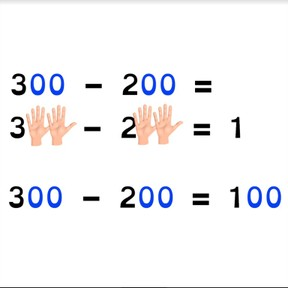Subtraction to 1,000 with the zero-rule

# Subtraction to 1,000 with the zero-rule

No account needed.8,000 schools use Gynzy92,000 teachers use Gynzy1,600,000 students use Gynzy

## General

Students learn to subtract with hundreds to 100 with the zero rule. They learn to easily subtract hundreds by making use of a first step without zeros.

2.NBT.B.5

## Relevance

When students are able to subtract numbers with the zero rule, they are able to subtract hundreds quickly and easily..

## Introduction

The interactive whiteboard shows 50 MAB blocks in five sticks of ten. Ask the students how many there are and drag 30 to the box. Ask how many sticks of 10 they have dragged to the box. Then show 500 MAB blocks in cubes of one hundred. Ask students how many there are and drag 300 to the box. Ask students how many cubes they dragged to the box.

## Development

Discuss with the class that it is important to learn what the zero rule is so that you can quickly and easily solve subtraction problems with hundreds. Remind students that tens always end with a zero in the ones place and that hundreds always end with a zero in the ones and the tens place. Show students the zero rule with the problems 2 - 1, 20 - 10, 200 - 100 with MAB blocks on the interactive whiteboard so that students have a visual impression of what the first step of these subtraction problems would be. Explain the zero rule with an example. Show students that if you have a subtraction problem with hundreds numbers, that you can take a first step by solving the subtraction problem without the zeros. You make the subtraction problem 100 times as small. When you have two zeros (in the tens and ones places) in both numbers, you have to add two zeros (in the tens and ones places) in the difference. Next explain that if you have the first step, you can use that because every zero you add makes the difference ten times as large. Practice three problems with the students using the zero rule, making sure that you ask students to name the first step, and how to name how many times larger the answer has become by adding the zeros to the tens or ones places.

Check that students are able to subtract numbers to 1,000 by asking the following questions:
-
- Which numbers do you add when using the zero rule?
- What do you do with thousands that you want to subtract easily?
- What happens with the difference if both numbers in the subtraction problem had zeros in the tens and ones places?

## Guided practice

Students start by solving a subtraction problem in which the difference of the first step is shown, and in following problems students must solve the first step themselves, and finally come up with the first step themselves.

## Closing

Check that students are able to subtract hundreds with the zero rule by asking them what they do with hundreds if you want to subtract these quickly. Ask why it is easy to be able to do this and ask them to demonstrate with 500 - 300. Then practice with three subtraction problems and ask students to drag the first step to the matching subtraction problems. Then solve the subtraction problems. Finally ask students to work in groups of three on subtraction problems with the zero rule. The first student writes the subtraction problem with hundreds, the second student writes the first step, and the third student solves the subtraction problem. Have them take turns so each gets a turn in each of the roles.

## Teaching tips

Students who have difficulty with subtracting numbers to 1,000 with the zero rule can be supported by working on some problems together. Walk through the steps with the students, discussing the creation of the first step of the subtraction problem without zeros.

## Instruction materials

Optional: paper and pencil

### The online teaching platform for interactive whiteboards and displays in schools

• Save time building lessons

• Manage the classroom more efficiently

• Increase student engagement